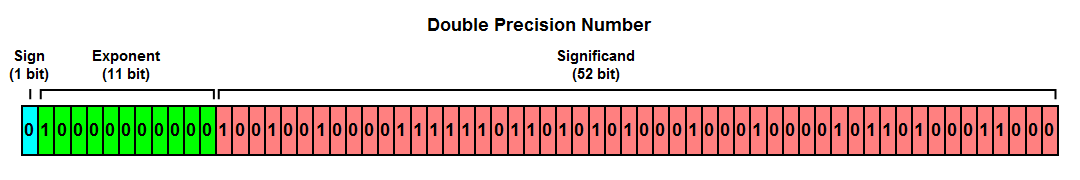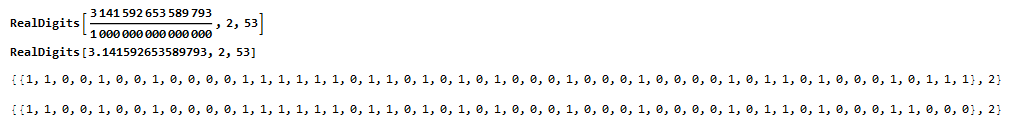# Digits Of Pi Needed For Floating Point Numbers

!WARNING DO NOT JUST USE THE MINIMUM AMOUNT LISTED IN THE TABLE. SEE CONCLUSION!

### Introduction

As one learns multiple programming languages one notices that basic mathematical constants such as pi are defined differently in in each language. Table 1 below gives the definition for pi for multiple popular programing languages:

 Language Command Digits of Pi Value Java Math.PI 16 3.141592653589793 C/C++ M_PI 21 3.14159265358979323846 Python math.pi 21 3.14159265358979323846 Swift 3.0 Double.PI >=16 3.141592653589793… Go Pi 63 3.141592653589793238462643383279

Table 1: List of popular programing languages and their definition of pi.

After seeing this spread the question quickly becomes, how many digits of pi are need? The answer depends on how the value of pi is stored.

### Floating point numbers

Decimal numbers are stored on computers by converting them to binary floating point numbers. These floating point numbers are defined in IEEE Standard for Floating-Point Arithmetic (IEEE 754). A floating point number is written out much the same way as a number written in scientific notation, which has a digits portion multiplied by an exponent. A floating point number is divided into three parts. A single sign bit which encodes the sign of the number. A significand (also called mantissa) which encodes the digits of the number and an exponent which is multiplied by the significand. Table 2 lists the floating point numbers defined by IEEE 754:

 Precision Type Total Bits Sign Exponent Significand Decimal Digits Half 16 1 5 10 ~3.31 Single 32 1 8 23 ~7.22 Double 64 1 11 52 ~15.95 Quadruple 128 1 15 112 ~34.02 Octuple 256 1 19 236 ~71.34

Table 2: Breakdown of bits used for sign, exponent and significand for different precision types of floating point numbers defined by IEEE 754.

Figure 1 shows what pi would look like if it was encoded in a double precision number.Figure 1: Double precision number comprised of a 1 bit sign field, 11 bit exponent field and a 52 bit significand field. In this case pi (3.141592653589793), has been encoded into the double precision floating point number. Note that the true value of this double precision number is 3.14159265358979311599796346854.

There are multiple ways to store a decimal number in binary with a varying level of precision. In nearly 99% of cases floats (single precision) and doubles (Double precision) are the typical ways most numbers are stored. Half precision numbers are typically used in GPU calculations such as video game lighting. Quadruple and octuplet precision are only encountered in extremely precise mathematical calculations such as physics simulations.

Now that we know how many bits (significand bits) we have to encode a decimal number we just now need to go through the process of converting the decimal number to a binary floating point number.

### Converting decimal to single precision floating point number

Correctly encoding a decimal number to a floating point number is hard. This is not due to the complexity but because any algorithm used to produce said conversion must be extremely fast and accurate.

1. Express decimal number as an integer times a power of ten.

$$3.141 \Rightarrow 3141*10^{-3}$$

2. Convert powers of ten into a fraction.

$$3141*10^{-3} \Rightarrow \frac{3141}{1000}$$

3. Scale into the range $$[2^{Significand},2^{Significand+1})$$

$$\frac{3141*2^{22}}{1000} \Rightarrow \frac{13174308864}{1000}$$

$$\frac{3141}{1000}$$ falls between $$2^{1}$$ and $$2^{2}$$. This number needs to be scaled to be between $$2^{Significand}$$ and $$2^{Significand+1}$$. The reason for the range going up to significand + 1 bits is that there is an implicit (hidden) leading bit to the significand that is always assumed to be 1. 0 is encoded by setting the exponent bits all to zero. Therefore the maximum amount of precision a floating point number has is significand bits + 1.

In this case we can multiply the numerator by $$2^{22}$$ to get it into the range needed for double precision numbers $$2^{23} \leq x < 2^{23+1}$$.

4. Divide and round

$$\frac{13174308864}{1000} \Rightarrow 13174308r 864$$

Dividing the numerator by the denominator, $$d$$, is now done resulting in the quotient, $$q$$ and the remainder, $$r$$. The quotient is an integer ranges between $$2^{Significand} \leq q < 2^{Significand+1}$$ and the remainder also an integer ranges between $$0 \leq r < d$$. The remainder is used to determine which way to round the quotient. IEEE 754 specifies that the rounding should be done using round half to even.

Round half to even follows these rules:

• $$r < \frac{d}{2}$$ round down
• $$r > \frac{d}{2}$$ round up
• $$r = \frac{d}{2}$$ round up if $$q$$ odd, round down if $$q$$ even.

In this case $$q=13174308$$ and $$r = 864$$. Since $$864> \frac{1000}{2}$$, $$q$$ is rounded up.

5. Convert to binary

$$13174309 = 110010010000011000100101$$

The rounded value for $$q$$ is then converted to a binary number of $$Significand+1$$ digits length.

6. Convert to scientific notation and reverse scaling

7. $$1.10010010000011000100101 * 2^{23} * 2^{-22}$$

$$1.10010010000011000100101 * 2^{1}$$

The binary number is written in normalized scientific notation (the decimal appears right after the first digit). It is then multiplied by the inverse of the previous applied scaling factor. The two exponents are then combined.

In this case since we are encoding a 24 digit binary number, the normalized scientific number has an exponent of $$2^{23}$$. The previous scaling of $$2^{22}$$ is then revered leading to the $$2^{-21}$$ term. Multiplying both exponents by one another results in the number being multiplies by $$2^{1}$$

8. Encode to floating point number

$$0100000001001001000001100010010011011101001011110001101010100$$

As previously stated floating point numbers are made up of three components. The sign, exponent and significand.

• The sign field is 0, since the number is positive
• $$2^{1}$$ is encoded in the exponent field as $$10000000$$
• The 23 bits after the decimal point are directly placed in the significand field.

### Minimum Amount Of Digits Need For Pi

Now that we have a method to convert decimal to a floating point binary number we can discover how many digits we need to accurately represent pi in each floating point precision type. This is done be adding digits to the representation of pi until the floating point value doesn’t change. Table 3 shows the result.

 Precision Type Digits Value Half 4 3.141 Single 8 3.14159265 Double 16 3.141592653589793 Quadruple 35 3.1415926535897932384626433832795028 Octuple 71 3.1415926535897932384626433832795028 841971693993751058209749445923078164

Table 3: Minimum decimal digits needed to accurately represent pi for a variety of floating point precision types. Warning: While these numbers represent the minimum decimal digits necessary you should use more digits.

There is no surprise in the numbers. Almost all are exactly in line with the decimal accuracy expected with floating point numbers. Since that is the case why does languages like C/C++ use 21 digits instead of 16?

### Conclusion

Table 3 tells the point at which adding more digits wont change the resulting floating point number. However, what these number doesn’t account for is what algorithm is used to convert these numbers from a decimal to a floating point binary number. As seen in Figure 2, even for programs specifically designed for computational precision (in this case Mathematica 11.0), different methods of representing the same decimal number might lead to different results.Figure 2: 16 digits of pi represented in both fractional notation and decimal notation converted to binary in Mathematica 11.0. Notice that the same number produces different binary results.

Due to this potential issues of being at the absolute minimum decimal digits, a few digits more should be added above the minimum. This is probably why most languages goes beyond the theoretical minimum number of decimal digits needed to accurately represent pi.